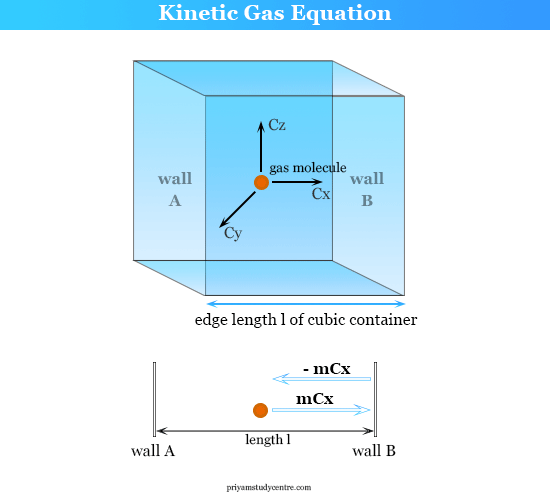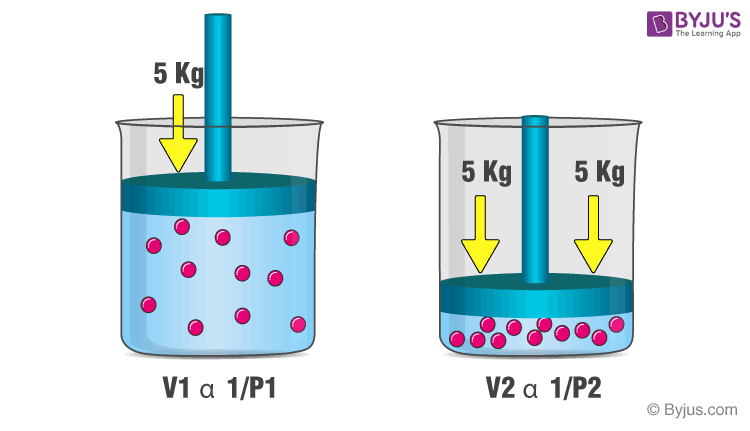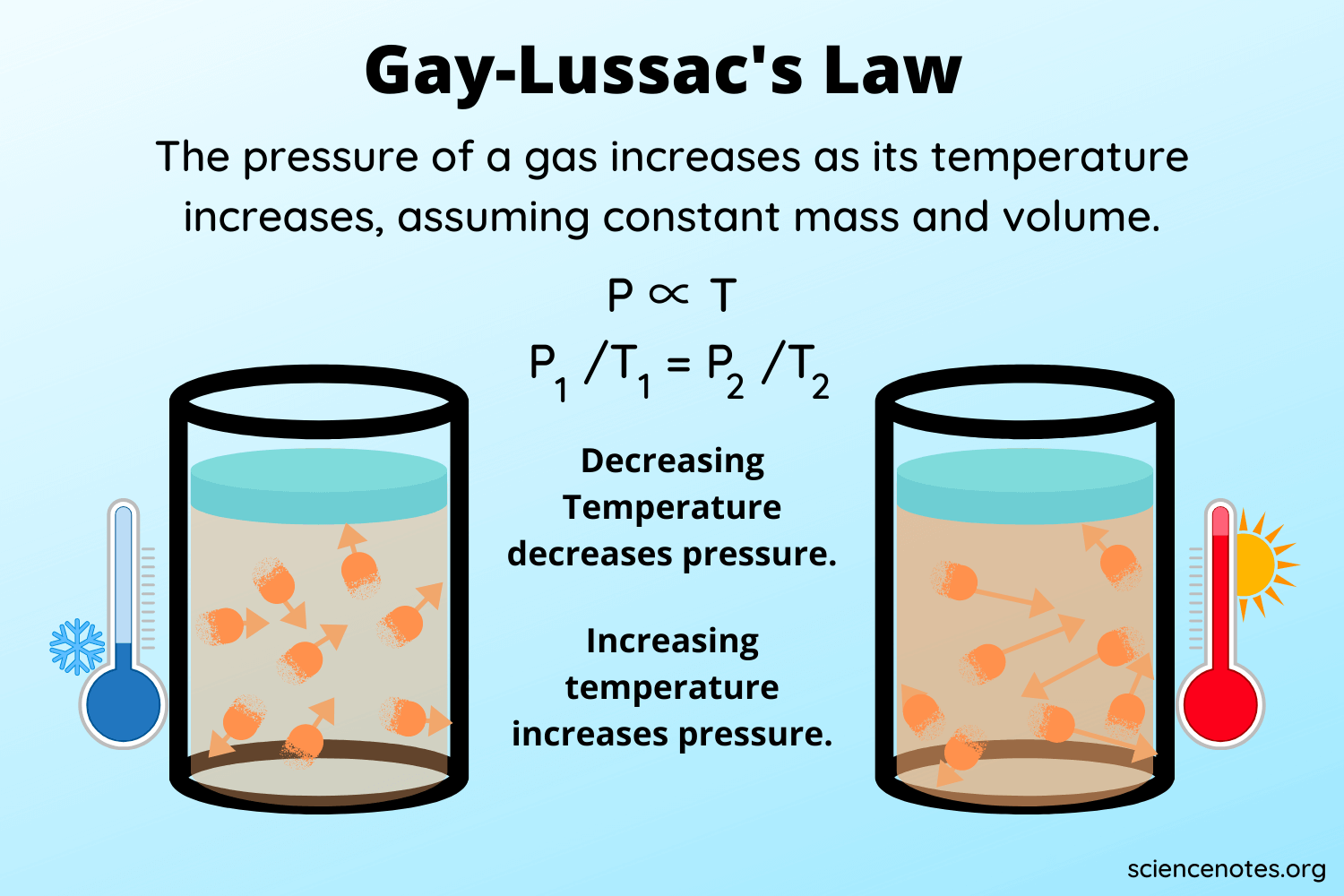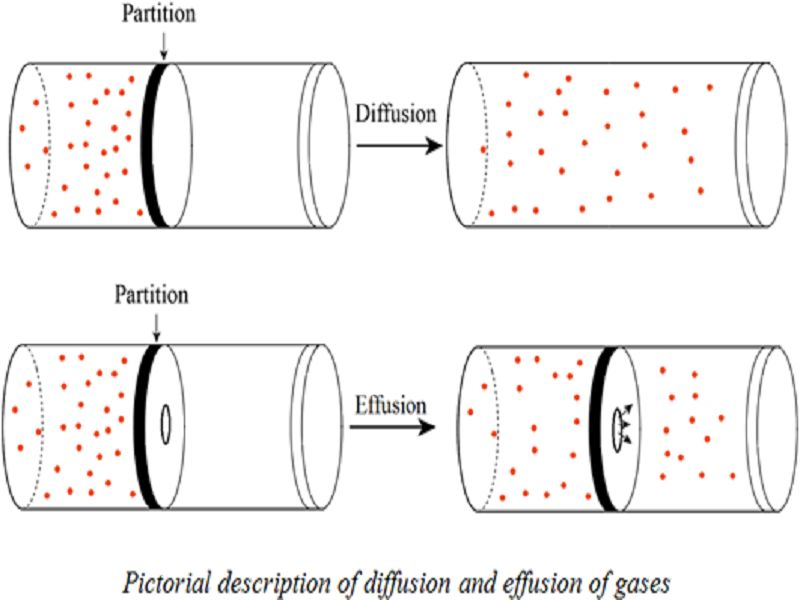# CBSE Class 11 Physics Chapter 13 Revision Notes

Chapter 13: Kinetic Theory Revision Notes

• Maxwell, Boltzman, and others developed the kinetic theory in the nineteenth century.
• The kinetic theory of gases explains how gases behave by assuming that the gas is made up of rapidly moving atoms or molecules.
• Optimum Gas: A perfect gas or ideal gas is one that strictly follows gas laws such as Boyle’s law, Charle’s law, Gay Lussac’s law, and so on.
• The characteristics of an ideal gas are as follows:

(i) An ideal gas molecule is a point mass that has no geometrical dimensions.

(ii) There are no forces of attraction or repulsion between the gas molecules.

Gas Pressure and Kinetic Theory

The pressure of a gas is the result of the gas molecules constantly bombarding the container’s walls. The pressure P exerted by an ideal gas is given bySource

Boyle’s Law

The volume (V) of a fixed mass of a gas is inversely proportional to the pressure (P) of the gas if the temperature of the gas is kept constant, according to this law.Source

Charles’s Law

The volume (V) of a given mass of a gas is directly proportional to the temperature of the gas if the pressure of the gas remains constant, according to this law.Source

Gay Lussac Law (or Pressure Law)

The pressure P of a given mass of a gas is directly proportional to its absolute temperature T, provided the volume V of the gas remains constant, according to this law.Source

Equation of State of a Gas

This is the relationship between pressure P, volume V, and absolute temperature T. The state equation of an ideal gas

PV = nRT,

where n is the number of moles of the enclosed gas and R is the universal molar gas constant, which is R = 8.315 JK-1 mob-1 for all gases.

Under S.T.P., equal volumes of all gases contain the same number of molecules, 6.023 x 1023.

• Graham’s Law of Gas Diffusion

The rate of diffusion of a gas is inversely proportional to the square root of the gas’s density, according to this formula.Source

As a result, the slower the rate of diffusion, the denser the gas.

Dalton’s Law of Partial Pressure

The resultant pressure exerted by a mixture of non-interacting gases is equal to the sum of their individual pressures, according to this law.

P = P1 + P2 + ————-Pn, for example.

• The arithmetic mean of the speeds of gas molecules is defined as the mean (or average) speed of gas molecules.

Temperature Kinetic Interpretation

The absolute temperature is proportional to the total average kinetic energy of all the molecules in a gas (T). As a result, a gas’s temperature is a measure of the average kinetic energy ‘IT of the gas’s molecules.

RT = 3/2 U

The average kinetic energy U is zero at T = 0, according to this interpretation of temperature, implying that molecular motion ceases completely at absolute zero.

• Degrees of Liberation

Degree of freedom refers to the total number of independent coordinates required to specify a molecule’s position or the number of independent modes of motion possible with any molecule.

The number of degrees of freedom in monoatomic, diatomic, and polyatomic (N) molecules is 3,5 or (3 N-K), where K is the number of constraints (structure-related restrictions).

• The Law of Energy Equipartition

The energy of a dynamic system in thermal equilibrium is distributed evenly among the various degrees of freedom, and the energy associated with each degree of freedom per molecule is 1/2 kT, where k is the Boltzman constant.

• Path of Least Resistance

The average distance traveled by a molecule in a gas between two successive collisions is known as its mean free path.

]]>